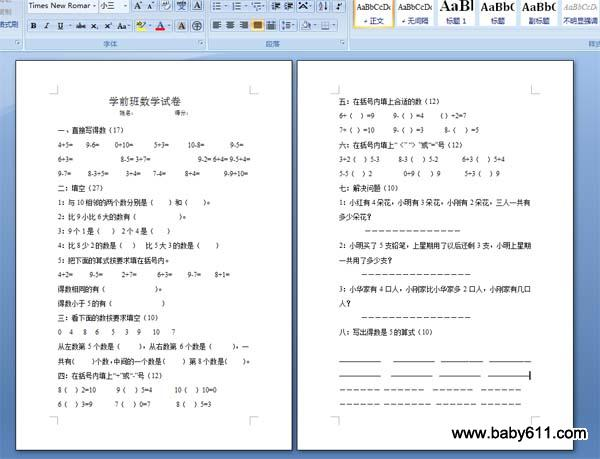# 学前班数学试卷-测试题一、直接写得数（17）4+5=      9-6=       0+10=         5+3=        10-8=            9-5=6+3= 8-5= 3+7= 9-2= 6+4= 9-5+4= 9-7=        8-3+5=         3+4=      7-4=        8+4=       9-9+10=二：填空（27）1：与10相邻的两个数分别是（       ）和（       ）。

2：比9小比6大的数有（                       ）。

3：9个1是（     ） 2个4是（      ）4：比8少2的数是（    ）   比5大3的数是（       ）5：把下面的算式按要求填在括号内。

4+2=        9-5=         2+7=          6+3=       9-7=      8+1=得数相同的有（                           ）。

得数小于5的有（                           ）三：看下面的数按要求填空（10）0    4     8    6      5     3    9      10      7从左数第5个数是（         ），从右数第6个数是（          ），一共有（        ）个数，中间的一个数是（        ） 第8个数是（       ）。

四：在括号内填上"+"或"-"号（12）8（   ）2=10          9（   ）5=4           10（   ）10=06（   ）3=9           7（   ）0=7             8（   ）5=3五：在括号内填上合适的数（12）6+（   ）=9         9-（ ）=4       （）+2=77+（ ）=10         9-（ ）=3          8-（   ）=5六：在括号内填上"〈" "〉"或"="号（12）3+2（   ）5-3         8-3（   ）5-2            6+3（   ）5+45-5（   ）2                0+9（    ）9            5+3（   ）9七：解决问题（10）1：小红有4朵花，小明有3朵花，小刚有2朵花，三人一共有多少朵花？

--------------2：小明买了5支铅笔，上星期用了以后还剩3支，小明上星期一共用了多少支？

----------------3：小华家有4口人，小刚家比小华家多2口人，小刚家有几口人？

此试卷格式为：WORD DOC格式，纸张：A4，页数：2页。

• 幼儿园试卷分类
• 将此试卷分享到：
• 数学最新更新